• 设计要求： 能滤除10Hz以下的低频信号 T = 32/1000; % 采样周期，s Fs = 1/T; % 采样频率，Hz % 注意：fp、fs值要小于Fs/2 fp = 8; % 通带截止频率，Hz fs = 12; % 阻带截止频率，Hz wp = 2*pi*fp/Fs...
设计要求：

能滤除10Hz以下的低频信号

T  = 32/1000;       % 采样周期，s
Fs = 1/T;           % 采样频率，Hz

% 注意：fp、fs值要小于Fs/2
fp = 8;          					% 通带截止频率，Hz
fs = 12;         					% 阻带截止频率，Hz
wp = 2*pi*fp/Fs;  					% 转换单位并归一化处理
ws = 2*pi*fs/Fs;
Rp = 3;           					% 通带允许最大衰减，db
Rs = 30;          					% 阻带允许最小衰减，db
[n,Wn]=cheb1ord(wp/pi,ws/pi,Rp,Rs); % 获取阶数和截止频率
[b,a]=cheby1(n,Rp,Wn, 'high')  		% 获得转移函数系数

% 滤波器查看
[H,w]=freqz(b,a);
plot(w*Fs/(2*pi),20*log10(abs(H)));
axis([0 20 -30 0]);
ylabel('增益/dB');xlabel('频率（Hz）');title('数字滤波器幅度响应');grid on;

% 滤波器使用
%dataOut = filter(b,a,dataIn);          %信号滤波运算



展开全文• fc=0.3KHz，δ=0.8dB，fr=0.2KHz，At=20dB，T=1ms；设计一个切比雪夫高通滤波器，观察其通带损耗和阻带衰减是否满足要求
• 我想很多学习电子和通信的朋友一定对于MATLAB很是熟悉，做课程设计的时候会需要一些经典的源程序来进行学习和修改，现在就提供一个基于双线性变换的切比雪夫II型高通滤波器设计源代码欢迎大家下载学习。
• 要求 有源高通滤波器，在100Hz处衰减3dB，在25Hz处最小衰减为75dB。 　解 ①计算高通滤波器的陡度系数：   　②首先选择归一化低通滤波器，使其在频率变换比4：1的范围内，衰减由3dB变换为75dB。图1所示曲线表明...
• 线性卷积和循环卷积；模拟采样定理的实现；切比雪夫I型低通滤波器设计； 凯塞窗设计数字高通滤波器matlab
• 切比雪夫Ⅰ型滤波器特点：1、幅度特性是在一个...测试代码：% Cheby1Filter.m% 切比雪夫Ⅰ型滤波器设计%clear;close all;clc;fs = 1000; %Hz 采样频率Ts = 1/fs;N = 1000; %序列长度t = (0:N-1)*Ts;delta_f = 1*...
切比雪夫Ⅰ型滤波器特点：1、幅度特性是在一个频带内(通带或阻带)范围内具有等波纹特性；2、Ⅰ型在通带范围内是等波纹的，在阻带范围内是单调的。测试代码：%  Cheby1Filter.m%  切比雪夫Ⅰ型滤波器的设计%clear;close all;clc;fs = 1000; %Hz 采样频率Ts = 1/fs;N  = 1000; %序列长度t = (0:N-1)*Ts;delta_f = 1*fs/N;f1 = 50;f2 = 100;f3 = 200;f4 = 400;x1 = 2*0.5*sin(2*pi*f1*t);x2 = 2*0.5*sin(2*pi*f2*t);x3 = 2*0.5*sin(2*pi*f3*t);x4 = 2*0.5*sin(2*pi*f4*t);x = x1 + x2 + x3 + x4; %待处理信号由四个分量组成X = fftshift(abs(fft(x)))/N;X_angle = fftshift(angle(fft(x)));f = (-N/2:N/2-1)*delta_f;figure(1);subplot(3,1,1);plot(t,x);title('原信号');subplot(3,1,2);plot(f,X);grid on;title('原信号频谱幅度特性');subplot(3,1,3);plot(f,X_angle);title('原信号频谱相位特性');grid on;%设计一个切比雪夫低通滤波器,要求把50Hz的频率分量保留,其他分量滤掉wp = 55/(fs/2);  %通带截止频率,取50~100中间的值,并对其归一化ws = 90/(fs/2);  %阻带截止频率,取50~100中间的值,并对其归一化alpha_p = 3; %通带允许最大衰减为 dbalpha_s = 40;%阻带允许最小衰减为 db%获取阶数和截止频率[ N1 wc1 ] = cheb1ord( wp , ws , alpha_p , alpha_s);%获得转移函数系数[ b a ] = cheby1(N1,alpha_p,wc1,'low');%滤波filter_lp_s = filter(b,a,x);X_lp_s = fftshift(abs(fft(filter_lp_s)))/N;X_lp_s_angle = fftshift(angle(fft(filter_lp_s)));figure(2);freqz(b,a); %滤波器频谱特性figure(3);subplot(3,1,1);plot(t,filter_lp_s);grid on;title('低通滤波后时域图形');subplot(3,1,2);plot(f,X_lp_s);title('低通滤波后频域幅度特性');subplot(3,1,3);plot(f,X_lp_s_angle);title('低通滤波后频域相位特性');%设计一个高通滤波器,要求把400Hz的频率分量保留,其他分量滤掉wp = 350/(fs/2);  %通带截止频率,取200~400中间的值,并对其归一化ws = 380/(fs/2);  %阻带截止频率,取200~400中间的值,并对其归一化alpha_p = 3; %通带允许最大衰减为  dbalpha_s = 20;%阻带允许最小衰减为  db%获取阶数和截止频率[ N2 wc2 ] = cheb1ord( wp , ws , alpha_p , alpha_s);%获得转移函数系数[ b a ] = cheby1(N2,alpha_p,wc2,'high');%滤波filter_hp_s = filter(b,a,x);X_hp_s = fftshift(abs(fft(filter_hp_s)))/N;X_hp_s_angle = fftshift(angle(fft(filter_hp_s)));figure(4);freqz(b,a); %滤波器频谱特性figure(5);subplot(3,1,1);plot(t,filter_hp_s);grid on;title('高通滤波后时域图形');subplot(3,1,2);plot(f,X_hp_s);title('高通滤波后频域幅度特性');subplot(3,1,3);plot(f,X_hp_s_angle);title('高通滤波后频域相位特性');%设计一个带通滤波器,要求把50Hz和400Hz的频率分量滤掉,其他分量保留wp = [65 385 ] / (fs/2);  %通带截止频率,50~100、200~400中间各取一个值,并对其归一化ws = [75 375 ] / (fs/2);  %阻带截止频率,50~100、200~400中间各取一个值,并对其归一化alpha_p = 3; %通带允许最大衰减为  dbalpha_s = 20;%阻带允许最小衰减为  db%获取阶数和截止频率[ N3 wn ] = cheb1ord( wp , ws , alpha_p , alpha_s);%获得转移函数系数[ b a ] = cheby1(N3,alpha_p,wn,'bandpass');%滤波filter_bp_s = filter(b,a,x);X_bp_s = fftshift(abs(fft(filter_bp_s)))/N;X_bp_s_angle = fftshift(angle(fft(filter_bp_s)));figure(6);freqz(b,a); %滤波器频谱特性figure(7);subplot(3,1,1);plot(t,filter_bp_s);grid on;title('带通滤波后时域图形');subplot(3,1,2);plot(f,X_bp_s);title('带通滤波后频域幅度特性');subplot(3,1,3);plot(f,X_bp_s_angle);title('带通滤波后频域相位特性');%设计一个带阻滤波器,要求把50Hz和400Hz的频率分量保留,其他分量滤掉wp = [65 385 ] / (fs/2);  %通带截止频率?,50~100、200~400中间各取一个值,并对其归一化ws = [75 375 ] / (fs/2);  %阻带截止频率?,50~100、200~400中间各取一个值,并对其归一化alpha_p = 3; %通带允许最大衰减为  dbalpha_s = 20;%阻带允许最小衰减为  db%获取阶数和截止频率[ N4 wn ] = cheb1ord( wp , ws , alpha_p , alpha_s);%获得转移函数系数[ b a ] = cheby1(N4,alpha_p,wn,'stop');%滤波filter_bs_s = filter(b,a,x);X_bs_s = fftshift(abs(fft(filter_bs_s)))/N;X_bs_s_angle = fftshift(angle(fft(filter_bs_s)));figure(8);freqz(b,a); %滤波器频谱特性figure(9);subplot(3,1,1);plot(t,filter_bs_s);grid on;title('带阻滤波后时域图形');subplot(3,1,2);plot(f,X_bs_s);title('带阻滤波后频域幅度特性');subplot(3,1,3);plot(f,X_bs_s_angle);title('带阻滤波后频域相位特性');效果：原始信号：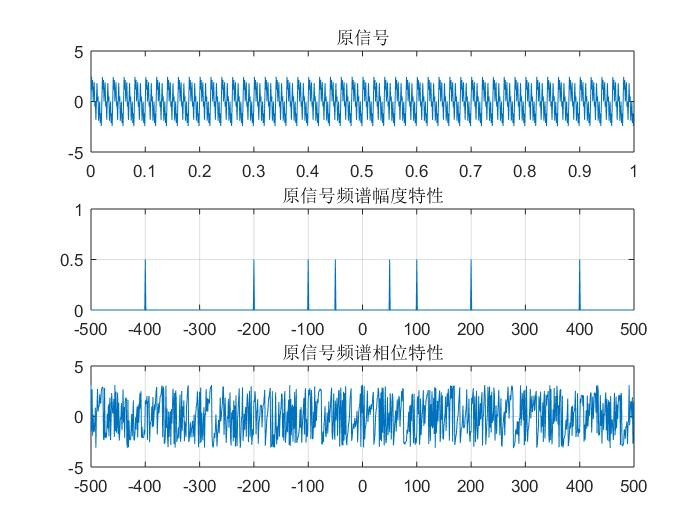生成的低通滤波器和滤波后的效果：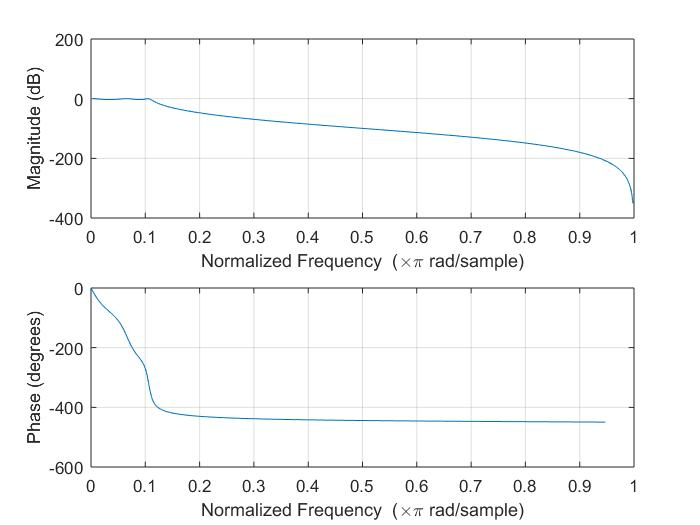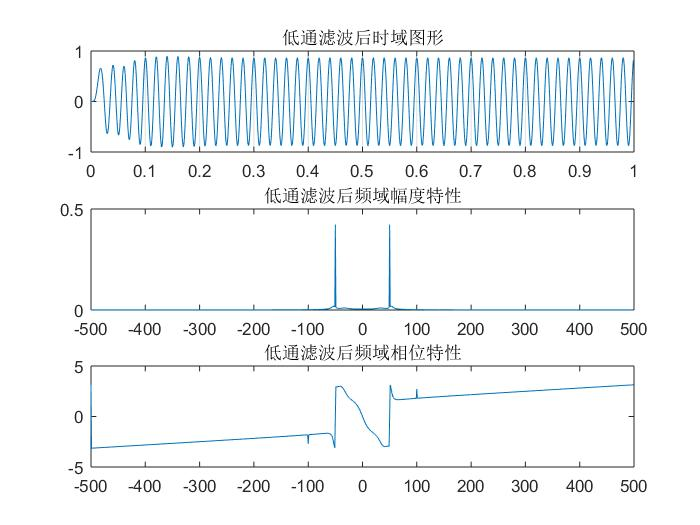生成的高通滤波器和滤波后的结果：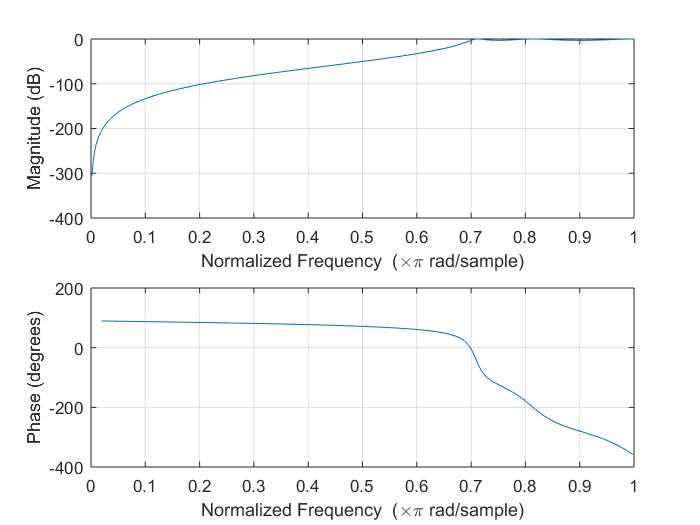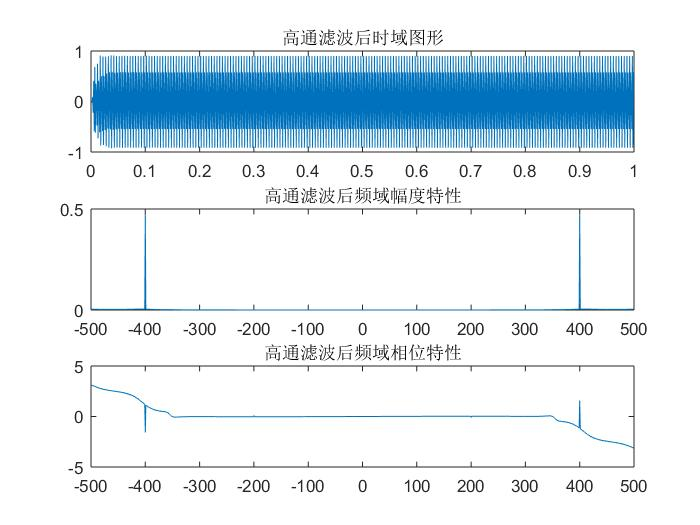生成的带通滤波器和滤波后的结果：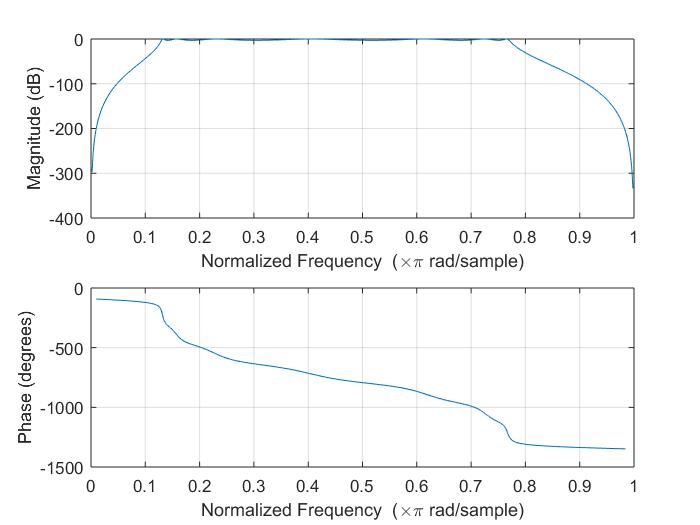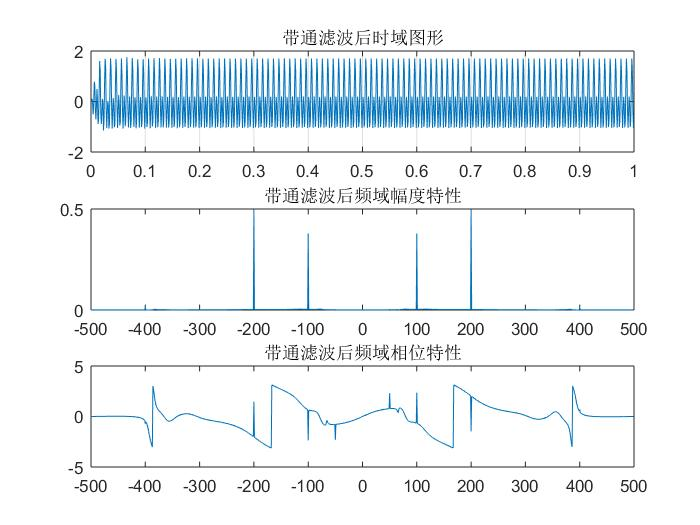生成的带阻滤波器和滤波后的结果：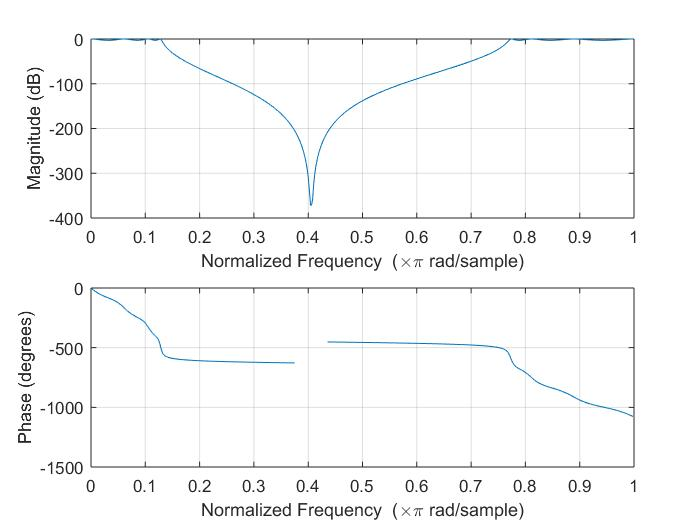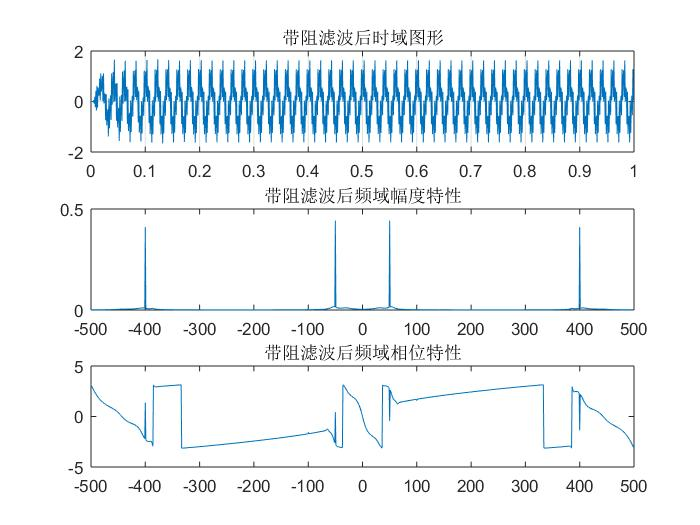展开全文• 简单记录下在matlab上如何设计出模拟的高通滤波器，包括：巴特沃斯滤波器、切比雪夫I型滤波器、切比雪夫II型滤波器、椭圆型滤波器。 %设计高通滤波器 %巴特沃斯、切比雪夫I型、切比雪夫II型、椭圆型滤波器 ...
简单记录下在matlab上如何设计出模拟的高通滤波器，包括：巴特沃斯滤波器、切比雪夫I型滤波器、切比雪夫II型滤波器、椭圆型滤波器。
%设计高通滤波器
%巴特沃斯、切比雪夫I型、切比雪夫II型、椭圆型滤波器

clear all;

%wp和ws分别是通带和阻带的频率(截止频率)。当wp和ws为二元矢量时，为带通或带阻滤波器，这是求出的Wn也是二元矢量
%当wp和ws为一元矢量时：当wp>ws为高通滤波器，当wp<ws为低通滤波器

%wp和ws为一元矢量
wp=0.1*2*pi;                            %设置通带频率
ws=0.05*2*pi;                           %设置阻带频率

Rp=1;                                   %设置通带波纹系数
Rs=20;                                  %设置阻带波纹系数

%巴特沃斯滤波器设计
[N,Wn]=buttord(wp,ws,Rp,Rs,'s');        %求巴特沃斯滤波器阶数，输出参数N代表满足设计要求的滤波器的最小阶数，Wn是等效低通滤波器的截止频率
%无论是高通、带通和带阻滤波器，在设计中最终都等效于一个截止频率为Wn的低通滤波器(我现在也不是很理解为啥是这样，毕竟我也是刚接触滤波器)
fprintf('巴特沃斯滤波器 N= %4d\n',N);    %显示滤波器阶数
[bb,ab]=butter(N,Wn,'high','s');        %求巴特沃斯滤波器系数，即求传输函数的分子和分母的系数向量
W=0:0.01:2;                             %设置模拟频率
[Hb,wb]=freqs(bb,ab,W);                 %求巴特沃斯滤波器频率响应
plot(wb/pi,20*log10(abs(Hb)),'b');      %作图
hold on

%切比雪夫I型滤波器设计
[N,Wn]=cheb1ord(wp,ws,Rp,Rs,'s');       %求切比雪夫I型滤波器阶数
fprintf('切比雪夫I型滤波器 N= %4d\n',N); %显示滤波器阶数
[bc1,ac1]=cheby1(N,Rp,Wn,'high','s');    %求切比雪夫I型滤波器系数，即求传输函数的分子和分母的系数向量
[Hc1,wc1]=freqs(bc1,ac1,W);             %求切比雪夫I型滤波器频率响应
plot(wc1/pi,20*log10(abs(Hc1)),'k');    %作图

%切比雪夫II型滤波器设计
[N,Wn]=cheb2ord(wp,ws,Rp,Rs,'s');       %求切比雪夫II型滤波器阶数
fprintf('切比雪夫II型滤波器 N= %4d\n',N);%显示滤波器阶数
[bc2,ac2]=cheby2(N,Rs,Wn,'high','s');    %求切比雪夫II型滤波器系数，即求传输函数的分子和分母的系数向量
[Hc2,wc2]=freqs(bc2,ac2,W);             %求切比雪夫II型滤波器频率响应
plot(wc2/pi,20*log10(abs(Hc2)),'r');    %作图

%椭圆型滤波器设计
[N,Wn]=ellipord(wp,ws,Rp,Rs,'s');       %求椭圆型滤波器阶数
fprintf('椭圆型滤波器 N= %4d\n',N);      %显示滤波器阶数
[be,ae]=ellip(N,Rp,Rs,Wn,'high','s');    %求椭圆型滤波器系数，即求传输函数的分子和分母的系数向量
[He,we]=freqs(be,ae,W);                 %求椭圆型滤波器频率响应
%作图
plot(we/pi,20*log10(abs(He)),'g');
axis([0 max(we/pi) -30 2]);
legend('巴特沃斯滤波器','切比雪夫I型滤波器','切比雪夫II型滤波器','椭圆型滤波器');
xlabel('角频率{\omega}/{\pi}');
ylabel('幅值/dB');
line([0 max(we/pi)],[-20 -20],'color','k','linestyle','--');
line([0 max(we/pi)],[-1 -1],'color','k','linestyle','--');
line([0.2 0.2],[-30 2],'color','k','linestyle','--');

运行结果如下：
巴特沃斯滤波器 N=    5
切比雪夫I型滤波器 N=    3
切比雪夫II型滤波器 N=    3
椭圆型滤波器 N=    3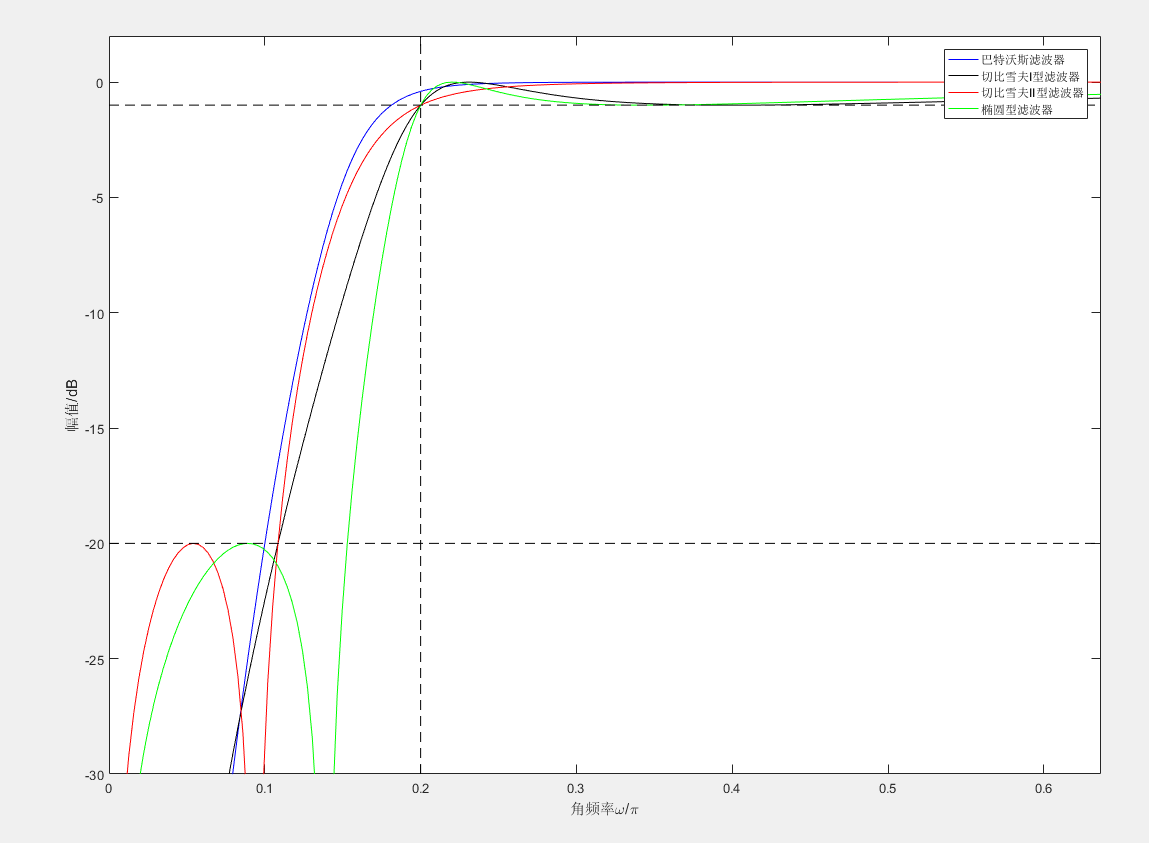展开全文• 下两个滤波器都是切比雪夫I型数字滤波器，不是巴特沃尔滤波器，请使用者注意！1.低通滤波器使用说明：将下列代码幅值然后以m文件保存，文件名要与函数名相同，这里函数名：lowp。function y=lowp(x,f1,f3,rp,rs,Fs) ...
本文为转载内容，原文地址为点击打开链接。下两个滤波器都是切比雪夫I型数字滤波器，不是巴特沃尔滤波器，请使用者注意！1.低通滤波器使用说明：将下列代码幅值然后以m文件保存，文件名要与函数名相同，这里函数名：lowp。function y=lowp(x,f1,f3,rp,rs,Fs)
%低通滤波
%使用注意事项：通带或阻带的截止频率的选取范围是不能超过采样率的一半
%即，f1,f3的值都要小于 Fs/2
%x:需要带通滤波的序列
% f 1：通带截止频率
% f 3：阻带截止频率
%rp：边带区衰减DB数设置
%rs：截止区衰减DB数设置
%FS：序列x的采样频率
% rp=0.1;rs=30;%通带边衰减DB值和阻带边衰减DB值
% Fs=2000;%采样率
%
wp=2*pi*f1/Fs;
ws=2*pi*f3/Fs;
% 设计切比雪夫滤波器；
[n,wn]=cheb1ord(wp/pi,ws/pi,rp,rs);
[bz1,az1]=cheby1(n,rp,wp/pi);
%查看设计滤波器的曲线
[h,w]=freqz(bz1,az1,256,Fs);
h=20*log10(abs(h));
figure;plot(w,h);title('所设计滤波器的通带曲线');grid on;
%
y=filter(bz1,az1,x);%对序列x滤波后得到的序列y
end--------------------------------------低通滤波器使用例子的代码fs=2000;
t=(1:fs)/fs;
ff1=100;
ff2=400;
x=sin(2*pi*ff1*t)+sin(2*pi*ff2*t);
figure;
subplot(211);plot(t,x);
subplot(212);hua_fft(x,fs,1);
%低通测试
% y=filter(bz1,az1,x);
y=lowp(x,300,350,0.1,20,fs);
figure;
subplot(211);plot(t,y);
subplot(212);hua_fft(y,fs,1);%hua_fft()函数是画频谱图的函数，代码在下面给出，要保存为m文件调用%这段例子还调用了我自己写的专门画频谱图的函数，也给出，不然得不出我的结果;%画信号的幅频谱和功率谱
%频谱使用matlab例子表示
function hua_fft(y,fs,style,varargin)
%当style=1,画幅值谱；当style=2,画功率谱;当style=其他的，那么花幅值谱和功率谱
%当style=1时，还可以多输入2个可选参数
%可选输入参数是用来控制需要查看的频率段的
%第一个是需要查看的频率段起点
%第二个是需要查看的频率段的终点
%其他style不具备可选输入参数，如果输入发生位置错误
nfft=2^nextpow2(length(y));%找出大于y的个数的最大的2的指数值（自动进算最佳FFT步长nfft）
%nfft=1024;%人为设置FFT的步长nfft
y=y-mean(y);%去除直流分量
y_ft=fft(y,nfft);%对y信号进行DFT，得到频率的幅值分布
y_p=y_ft.*conj(y_ft)/nfft;%conj()函数是求y函数的共轭复数，实数的共轭复数是他本身。
y_f=fs*(0:nfft/2-1)/nfft;�T变换后对应的频率的序列
% y_p=y_ft.*conj(y_ft)/nfft;%conj()函数是求y函数的共轭复数，实数的共轭复数是他本身。
if style==1
ifnargin==3
plot(y_f,2*abs(y_ft(1:nfft/2))/length(y));%matlab的帮助里画FFT的方法
%ylabel('幅值');xlabel('频率');title('信号幅值谱');
%plot(y_f,abs(y_ft(1:nfft/2)));%论坛上画FFT的方法
else
f1=varargin{1};
fn=varargin{2};
ni=round(f1 * nfft/fs+1);
na=round(fn * nfft/fs+1);
plot(y_f(ni:na),abs(y_ft(ni:na)*2/nfft));
end

elseif style==2
plot(y_f,y_p(1:nfft/2));
%ylabel('功率谱密度');xlabel('频率');title('信号功率谱');
else
subplot(211);plot(y_f,2*abs(y_ft(1:nfft/2))/length(y));
ylabel('幅值');xlabel('频率');title('信号幅值谱');
subplot(212);plot(y_f,y_p(1:nfft/2));
ylabel('功率谱密度');xlabel('频率');title('信号功率谱');
end
end下面三幅图分别是滤波前的时频图，滤波器的滤波特性曲线图和滤波后的时频图，通过图可以看出成功留下了100Hz的低频成分而把不要的高频成分去除了。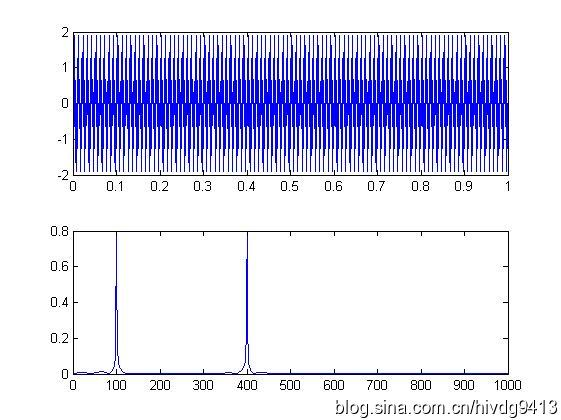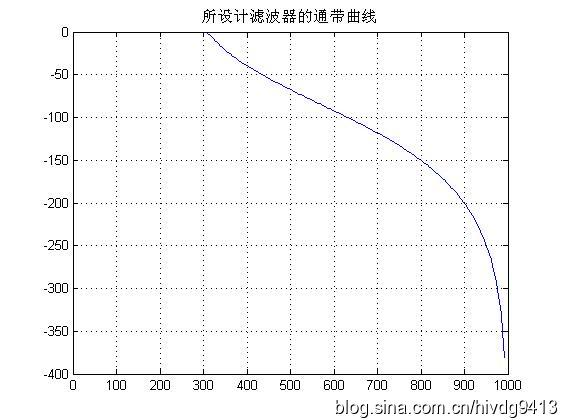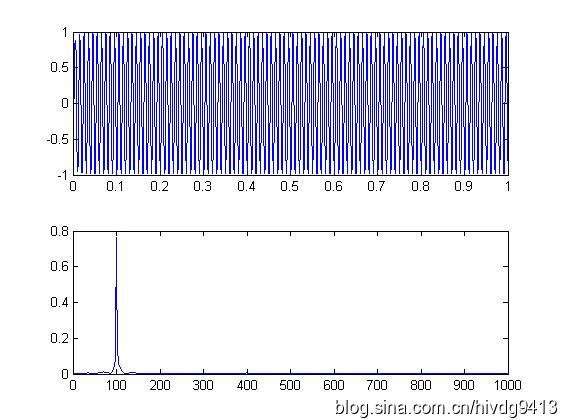2.高通滤波器function y=highp(x,f1,f3,rp,rs,Fs)
%高通滤波
%使用注意事项：通带或阻带的截止频率的选取范围是不能超过采样率的一半
%即，f1,f3的值都要小于 Fs/2
%x:需要带通滤波的序列
% f 1：通带截止频率
% f 2：阻带截止频率
%rp：边带区衰减DB数设置
%rs：截止区衰减DB数设置
%FS：序列x的采样频率
% rp=0.1;rs=30;%通带边衰减DB值和阻带边衰减DB值
% Fs=2000;%采样率
%
wp=2*pi*f1/Fs;
ws=2*pi*f3/Fs;
% 设计切比雪夫滤波器；
[n,wn]=cheb1ord(wp/pi,ws/pi,rp,rs);
[bz1,az1]=cheby1(n,rp,wp/pi,'high');

%查看设计滤波器的曲线
[h,w]=freqz(bz1,az1,256,Fs);
h=20*log10(abs(h));
figure;plot(w,h);title('所设计滤波器的通带曲线');grid on;
y=filter(bz1,az1,x);
end下面是高通滤波器的例子fs=2000;
t=(1:fs)/fs;
ff1=100;
ff2=400;
x=sin(2*pi*ff1*t)+sin(2*pi*ff2*t);
figure;
subplot(211);plot(t,x);
subplot(212);hua_fft(x,fs,1);

%------高通测试
z=highp(x,350,300,0.1,20,fs);
figure;
subplot(211);plot(t,z);
subplot(212);hua_fft(z,fs,1);下面三幅图分别是滤波前的时频图，滤波器的滤波特性曲线图和滤波后的时频图，通过图可以看出成功留下了400Hz的高频成分而把不要的低频成分100Hz去除了。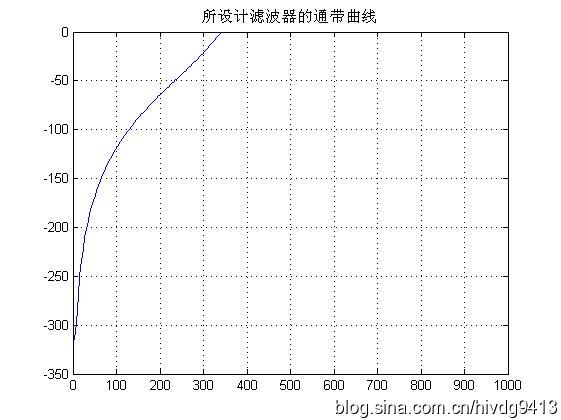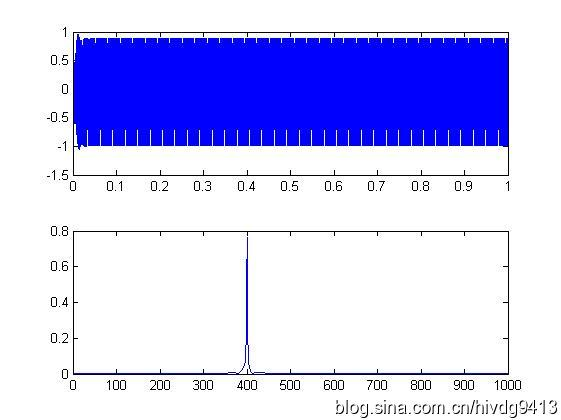3.带通滤波器function y=bandp(x,f1,f3,fsl,fsh,rp,rs,Fs)
%带通滤波
%使用注意事项：通带或阻带的截止频率与采样率的选取范围是不能超过采样率的一半
%即，f1,f3,fs1,fsh,的值小于 Fs/2
%x:需要带通滤波的序列
% f 1：通带左边界
% f 3：通带右边界
% fs1：衰减截止左边界
% fsh：衰变截止右边界
%rp：边带区衰减DB数设置
%rs：截止区衰减DB数设置
%FS：序列x的采样频率
% f1=300;f3=500;%通带截止频率上下限
% fsl=200;fsh=600;%阻带截止频率上下限
% rp=0.1;rs=30;%通带边衰减DB值和阻带边衰减DB值
% Fs=2000;%采样率
%
wp1=2*pi*f1/Fs;
wp3=2*pi*f3/Fs;
wsl=2*pi*fsl/Fs;
wsh=2*pi*fsh/Fs;
wp=[wp1 wp3];
ws=[wsl wsh];
%
% 设计切比雪夫滤波器；
[n,wn]=cheb1ord(ws/pi,wp/pi,rp,rs);
[bz1,az1]=cheby1(n,rp,wp/pi);
%查看设计滤波器的曲线
[h,w]=freqz(bz1,az1,256,Fs);
h=20*log10(abs(h));
figure;plot(w,h);title('所设计滤波器的通带曲线');grid on;
y=filter(bz1,az1,x);
end带通滤波器使用例子%--------------
%带通滤波器测试程序
fs=2000;
t=(1:fs)/fs;
ff1=100;
ff2=400;
ff3=700;
x=sin(2*pi*ff1*t)+sin(2*pi*ff2*t)+sin(2*pi*ff3*t);
figure;
subplot(211);plot(t,x);
subplot(212);hua_fft(x,fs,1);
% y=filter(bz1,az1,x);
y=bandp(x,300,500,200,600,0.1,30,fs);
figure;
subplot(211);plot(t,y);
subplot(212);hua_fft(y,fs,1);%调用到的hua_fft()函数代码如下function hua_fft(y,fs,style,varargin)
%当style=1,画幅值谱；当style=2,画功率谱;当style=其他的，那么花幅值谱和功率谱
%当style=1时，还可以多输入2个可选参数
%可选输入参数是用来控制需要查看的频率段的
%第一个是需要查看的频率段起点
%第二个是需要查看的频率段的终点
%其他style不具备可选输入参数，如果输入发生位置错误
nfft=2^nextpow2(length(y));%找出大于y的个数的最大的2的指数值（自动进算最佳FFT步长nfft）
%nfft=1024;%人为设置FFT的步长nfft
y=y-mean(y);%去除直流分量
y_ft=fft(y,nfft);%对y信号进行DFT，得到频率的幅值分布
y_p=y_ft.*conj(y_ft)/nfft;%conj()函数是求y函数的共轭复数，实数的共轭复数是他本身。
y_f=fs*(0:nfft/2-1)/nfft;�T变换后对应的频率的序列
% y_p=y_ft.*conj(y_ft)/nfft;%conj()函数是求y函数的共轭复数，实数的共轭复数是他本身。
if style==1
ifnargin==3
plot(y_f,2*abs(y_ft(1:nfft/2))/length(y));%matlab的帮助里画FFT的方法
%ylabel('幅值');xlabel('频率');title('信号幅值谱');
%plot(y_f,abs(y_ft(1:nfft/2)));%论坛上画FFT的方法
else
f1=varargin{1};
fn=varargin{2};
ni=round(f1 * nfft/fs+1);
na=round(fn * nfft/fs+1);
plot(y_f(ni:na),abs(y_ft(ni:na)*2/nfft));
end

elseif style==2
plot(y_f,y_p(1:nfft/2));
%ylabel('功率谱密度');xlabel('频率');title('信号功率谱');
else
subplot(211);plot(y_f,2*abs(y_ft(1:nfft/2))/length(y));
ylabel('幅值');xlabel('频率');title('信号幅值谱');
subplot(212);plot(y_f,y_p(1:nfft/2));
ylabel('功率谱密度');xlabel('频率');title('信号功率谱');
end
end运行结果如下图，第一幅是滤波前测试信号的时频图，第二幅是滤波器的滤波曲线图，第三幅是经滤波后的测试信号时频图。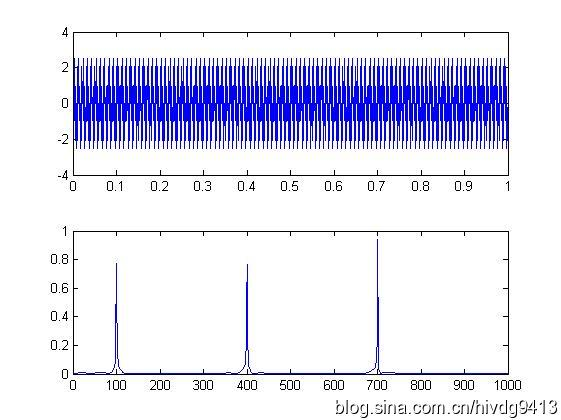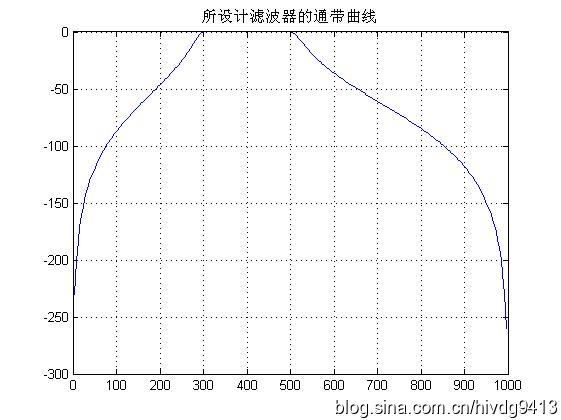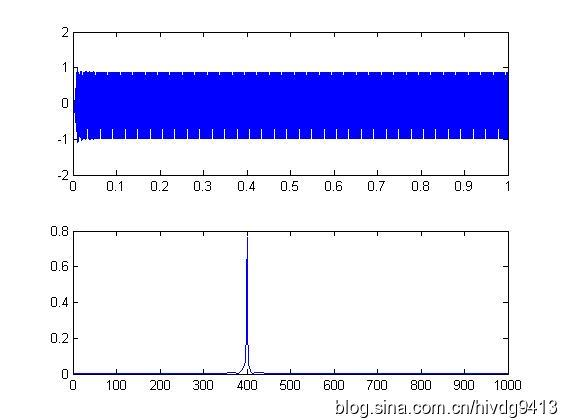4.带阻滤波器function y=bands(x,f1,f3,fsl,fsh,rp,rs,Fs)
%带阻滤波
%使用注意事项：通带或阻带的截止频率与采样率的选取范围是不能超过采样率的一半
%即，f1,f3,fs1,fsh,的值小于 Fs/2
%x:需要带通滤波的序列
% f 1：通带左边界
% f 3：通带右边界
% fs1：衰减截止左边界
% fsh：衰变截止右边界
%rp：边带区衰减DB数设置
%rs：截止区衰减DB数设置
%FS：序列x的采样频率
% f1=300;f3=500;%通带截止频率上下限
% fsl=200;fsh=600;%阻带截止频率上下限
% rp=0.1;rs=30;%通带边衰减DB值和阻带边衰减DB值
% Fs=2000;%采样率
%
wp1=2*pi*f1/Fs;
wp3=2*pi*f3/Fs;
wsl=2*pi*fsl/Fs;
wsh=2*pi*fsh/Fs;
wp=[wp1 wp3];
ws=[wsl wsh];
%
% 设计切比雪夫滤波器；
[n,wn]=cheb1ord(ws/pi,wp/pi,rp,rs);
[bz1,az1]=cheby1(n,rp,wp/pi,'stop');
%查看设计滤波器的曲线
[h,w]=freqz(bz1,az1,256,Fs);
h=20*log10(abs(h));
figure;plot(w,h);title('所设计滤波器的通带曲线');grid on;
y=filter(bz1,az1,x);
end使用例子%带阻滤波器测试
fs=1000;
t=(1:fs)/fs;
y=sin(2*pi*100*t)+sin(2*pi*150*t)+sin(2*pi*200*t);
figure;hua_fft(y,fs,1);
z=bands(y,110,190,140,160,0.1,30,fs);
figure;hua_fft(z,fs,1);运行结果如下图，第一幅是滤波前测试信号的频谱图，第二幅是滤波器的滤波曲线图，第三幅是经滤波后的测试信号频谱图。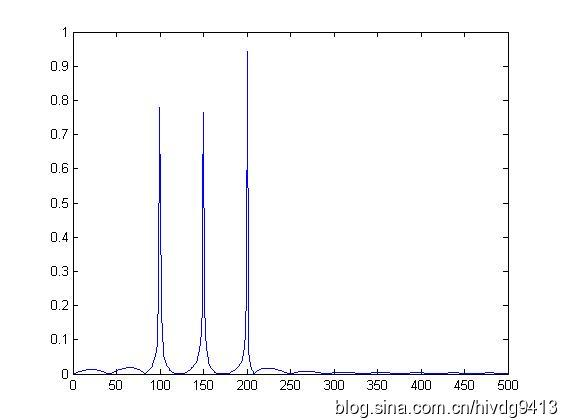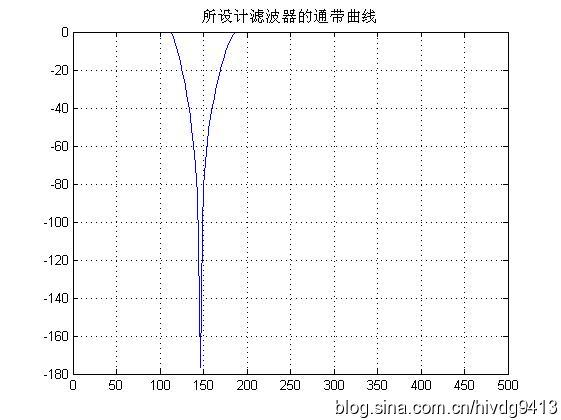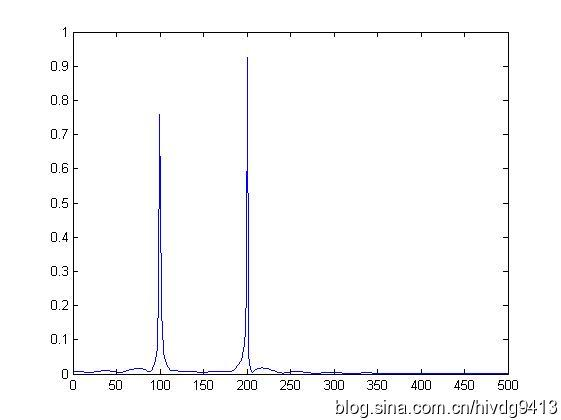展开全文低通滤波器 带通滤波器 带阻滤波器 matlab
• [Matlab]切比雪夫Ⅱ型滤波器设计：低通、高通、带通和带阻-------（3） II型切比雪夫滤波器 在阻带（或称“阻频带”）上频率响应幅度等波纹波动的滤波器称为“II型切比雪夫滤波器”。 也称倒数切比雪夫滤波器，较不...matlab 数字信号处理
• [Matlab]切比雪夫Ⅰ型滤波器设计：低通、高通、带通和带阻-------（2） ​ 切比雪夫滤波器，又名“车比雪夫滤波器”，是在通带或阻带上频率响应幅度等波纹波动的滤波器。切比雪夫滤波器来自切比雪夫分布，以...Matlab 信号处理
• 　③为了变换归一化低通滤波器为高通滤波器电路，可把电容、电感互相替换且元件值是原值的倒数。归一化高通滤波器如图2（b）所示。电感现在可以用图1所示的GIC电感替换，得到如图2（c）所示的高通滤波器
• 在MATLAB平台下，设计数字信号处理当中巴特沃斯和切比雪夫滤波器，包括低通、高通、带通和带阻等类型。
• 切比雪夫Ⅰ型滤波器特点： 1、幅度特性是在一个频带内（通带或阻带）范围内具有等波纹特性；...% 切比雪夫Ⅰ型滤波器设计 % clear; close all; clc; fs = 1000; %Hz 采样频率 Ts = 1/fs; N = 10...
• 切比雪夫Ⅱ型滤波器特点： 1、幅度特性是在一个频带内（通带或阻带）范围内具有等波纹特性；...% 切比雪夫Ⅱ型滤波器设计 % clear; close all; clc; fs = 1000; %Hz 采样频率 Ts = 1/fs; N = 10...
• 高通滤波器functiony=highp(x,f1,f3,rp,rs,Fs)%高通滤波%使用注意事项：通带或阻带的截止频率的选取范围是不能超过采样率的一半%即，f1,f3的值都要小于Fs/2%x:需要带通滤波的序列%f1：通带截止频率%f2：阻带截止频率...
• 要求 有源高通滤波器，在300Hz处衰减3士0.1dB，在l20Hz处最小衰减30dB，增益为2。 　解 ①计算高通陡度系数： 　②图1的曲线表明，一个3阶1dB切比雪夫滤波器在2.5rad/s处的衰减可以超过30dB。因为指标要求在截止...
• IIR一阶低通、高通、带通、带阻滤波器设计；以及North，ButterWorth滤波器实现，另外介绍了dolp-chebyshev滤波器的设计及应用
• 利用模拟低通滤波器设计带通、带阻、高通模拟滤波器 参考：《数字信号处理 第四版》高西全、丁玉美 最近学到第六章了，利用模拟低通滤波器设计不同类型的模拟滤波器，我们重点在设计巴特沃斯滤波器。 巴特沃斯滤波器...带阻滤波器 低通滤波器 带通滤波器
• 2" \h \z \u 1 课题综述 1 1.1 课题来源 1 1.2 预期目标 1 1.3 面对的问题 1 1.4 需要解决的关键技术 1 2 系统分析 2 2.1 涉及的基础知识 2 2.2 解决的基本思路 2 2.3 总体方案 2 2.4 功能模块框图 2 3 详细设计 ...